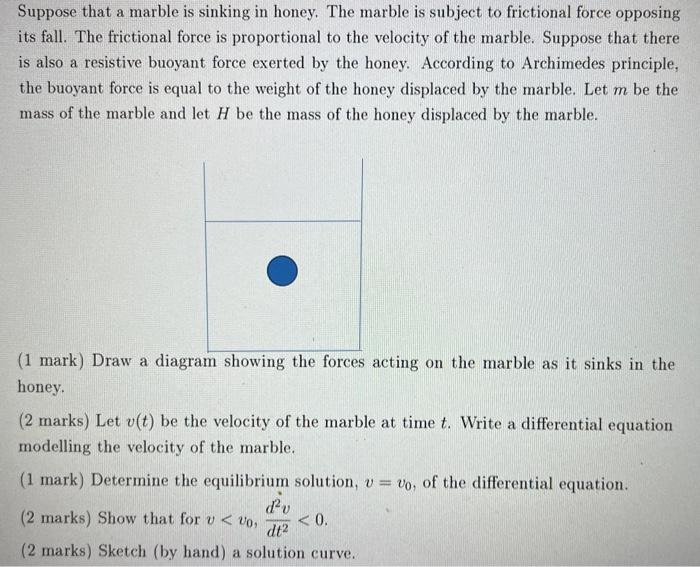# Question need help with this q Detailled ans with explanations will help Thanks  Suppose that a marble is sinking in honey. The marble is subject to frictional force opposing its fall. The frictional force is proportional to the velocity of the marble. Suppose that there is also a resistive buoyant force exerted by the honey. According to Archimedes principle, the buoyant force is equal to the weight of the honey displaced by the marble. Let $$m$$ be the mass of the marble and let $$H$$ be the mass of the honey displaced by the marble. (1 mark) Draw a diagram showing the forces acting on the marble as it sinks in the honey. ( 2 marks) Let $$v(t)$$ be the velocity of the marble at time $$t$$. Write a differential equation modelling the velocity of the marble. (1 mark) Determine the equilibrium solution, $$v=v_{0}$$, of the differential equation. (2 marks) Show that for $$v<v_{0}, \frac{d^{2} v}{d t^{2}}<0$$. (2 marks) Sketch (by hand) a solution curve.Transcribed Image Text: Suppose that a marble is sinking in honey. The marble is subject to frictional force opposing its fall. The frictional force is proportional to the velocity of the marble. Suppose that there is also a resistive buoyant force exerted by the honey. According to Archimedes principle, the buoyant force is equal to the weight of the honey displaced by the marble. Let $$m$$ be the mass of the marble and let $$H$$ be the mass of the honey displaced by the marble. (1 mark) Draw a diagram showing the forces acting on the marble as it sinks in the honey. ( 2 marks) Let $$v(t)$$ be the velocity of the marble at time $$t$$. Write a differential equation modelling the velocity of the marble. (1 mark) Determine the equilibrium solution, $$v=v_{0}$$, of the differential equation. (2 marks) Show that for $$v More Transcribed Image Text: Suppose that a marble is sinking in honey. The marble is subject to frictional force opposing its fall. The frictional force is proportional to the velocity of the marble. Suppose that there is also a resistive buoyant force exerted by the honey. According to Archimedes principle, the buoyant force is equal to the weight of the honey displaced by the marble. Let \( m$$ be the mass of the marble and let $$H$$ be the mass of the honey displaced by the marble. (1 mark) Draw a diagram showing the forces acting on the marble as it sinks in the honey. ( 2 marks) Let $$v(t)$$ be the velocity of the marble at time $$t$$. Write a differential equation modelling the velocity of the marble. (1 mark) Determine the equilibrium solution, $$v=v_{0}$$, of the differential equation. (2 marks) Show that for $$v Community AnswerM6XQHC &#12304;General guidance&#12305;The answer provided below has been developed in a clear step by step manner.Step1/3Diagram showing the forces acting on the marble as it sinks in the honey: ^ positive direction | |&lt;----Frictional force (Ff) |----&gt; |&lt;----Buoyant force (Fb) |----&gt; |&lt;----Weight of marble (mg) |----&gt; | v Let v(t) be the velocity of the marble at time t. The differential equation modelling the velocity of the marble is: \( \mathrm{{m}\times{d}\frac{{v}}{{\left.{d}{t}\right.}}={m}{g}-{F}{b}-{F}{f}}$$where m is the mass of the marble, g is the acceleration due to gravity, and Fb and Ff are the buoyant force and frictional force, respectively.Explanation:Please refer to solution in this step.Step2/3The equilibrium solution of the differential equation is found by setting $$\mathrm{{d}\frac{{v}}{{\left.{d}{t}\right.}}}$$ to zero:\( \mathrm{{m}{g}-{F}{b}- ... See the full answer